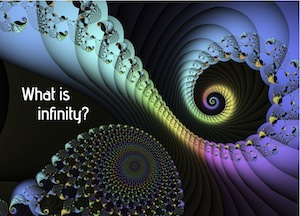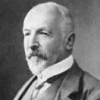# What is infinity?Image created by FAVIO.

Does infinity exist? Does it exist in the physical world or just in our heads? How many infinities are there? And are we allowed to do sums with them? Find out in these Plus articles.

What is infinity?What is infinity? Does it even exist? And how many infinities are there?

What is infinity?Take a trip into the never-ending.

Maths in a minute: Counting numbersAre there more irrational numbers than rational numbers, or more rational numbers than irrational numbers? Well, there are infinitely many of both, so the question doesn't make sense. It turns out, however, that the set of rational numbers is infinite in a very different way from the set of irrational numbers.

Do infinities exist in nature?Is the Universe finite or infinite? Is there infinity inside a black hole? Is space infinitely divisible or is there a shortest length? We talk to philosophers and physicists to find out.

Maths in a minute: Countable infinitiesAn infinite set is called countable if you can count it. In other words, it's called countable if you can put its members into one-to-one correspondence with the natural numbers 1, 2, 3, ... .
Does infinity exist: the podcastIn the latest poll of our Science fiction, science fact project you told us that you wanted to know if infinity exists. In this interview the cosmologist John D. Barrow gives us an overview on the question, from Aristotle's ideas to Cantor's never-ending tower of mathematical infinities, and from shock waves to black holes.

Does infinity exist?John Barrow gives us an overview, from Aristotle's ideas to Cantor's never-ending tower of mathematical infinities, and from shock waves to black holes.

Searching for the missing truthMany people like mathematics because it gives definite answers. Things are either true or false, and true things seem true in a very fundamental way. But it's not quite like that. You can actually build different versions of maths in which statements are true or false depending on your preference. So is maths just a game in which we choose the rules to suit our purpose? Or is there a "correct" set of rules to use? We find out with the mathematician Hugh Woodin.

Understanding uncertainty: Infinite monkey businessDavid Spiegelhalter explains that waiting for an infinite number of monkeys to produce the complete works of Shakespeare is not just a probabilistic certainty, it also gives us an insight into how long we can expect to wait for a rare event to happen.

Cantor and Cohen: Infinite investigators part IWhat's the nature of infinity? Are all infinities the same? And what happens if you've got infinitely many infinities? In this article Richard Elwes explores how these questions brought triumph to one man and ruin to another, ventures to the limits of mathematics and finds that, with infinity, you're spoilt for choice.
Cantor and Cohen: Infinite investigators part IIRichard Elwes continues his investigation into Cantor and Cohen's work. He investigates the continuum hypothesis, the question that caused Cantor so much grief.
A glimpse of Cantor's paradisePeter Macgregor explores the beautiful world of the infinite.
• Want facts and want them fast? Our Maths in a minute series explores key mathematical concepts in just a few words.